# modelDiscriminationPlot

Plot ROC curve

Since R2021b

## Syntax

``modelDiscriminationPlot(eadModel,data)``
``modelDiscriminationPlot(___,Name=Value)``
``h = modelDiscriminationPlot(ax,___,Name=Value)``

## Description

example

````modelDiscriminationPlot(eadModel,data)` generates the receiver operating characteristic (ROC) curve. `modelDiscriminationPlot` supports segmentation and comparison against a reference model.```

example

````modelDiscriminationPlot(___,Name=Value)` specifies options using one or more name-value arguments in addition to the input arguments in the previous syntax.```

example

````h = modelDiscriminationPlot(ax,___,Name=Value)` specifies options using one or more name-value arguments in addition to the input arguments in the previous syntax and returns the figure handle `h`.```

## Examples

collapse all

This example shows how to use `fitEADModel` to create a `Tobit` model and then use `modelDiscriminationPlot` to plot the ROC.

```load EADData.mat head(EADData)```
``` UtilizationRate Age Marriage Limit Drawn EAD _______________ ___ ___________ __________ __________ __________ 0.24359 25 not married 44776 10907 44740 0.96946 44 not married 2.1405e+05 2.0751e+05 40678 0 40 married 1.6581e+05 0 1.6567e+05 0.53242 38 not married 1.7375e+05 92506 1593.5 0.2583 30 not married 26258 6782.5 54.175 0.17039 54 married 1.7357e+05 29575 576.69 0.18586 27 not married 19590 3641 998.49 0.85372 42 not married 2.0712e+05 1.7682e+05 1.6454e+05 ```
```rng('default'); NumObs = height(EADData); c = cvpartition(NumObs,'HoldOut',0.4); TrainingInd = training(c); TestInd = test(c);```

Select Model Type

Select a model type for `Tobit` or `Regression`.

`ModelType =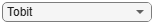"Tobit";`

Select Conversion Measure

Select a conversion measure for the EAD response values.

`ConversionMeasure =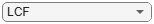"LCF";`

Create `Tobit` EAD Model

Use `fitEADModel` to create a `Tobit` model using the `TrainingInd` data.

```eadModel = fitEADModel(EADData(TrainingInd,:),ModelType,PredictorVars={'UtilizationRate','Age','Marriage'}, ... ConversionMeasure=ConversionMeasure,DrawnVar="Drawn",LimitVar="Limit",ResponseVar="EAD"); disp(eadModel);```
``` Tobit with properties: CensoringSide: "both" LeftLimit: 0 RightLimit: 1 ModelID: "Tobit" Description: "" UnderlyingModel: [1x1 risk.internal.credit.TobitModel] PredictorVars: ["UtilizationRate" "Age" "Marriage"] ResponseVar: "EAD" LimitVar: "Limit" DrawnVar: "Drawn" ConversionMeasure: "lcf" ```

Display the underlying model. The underlying model's response variable is the transformation of the EAD response data. Use the `'LimitVar'` and `'DrawnVar'` name-value arguments to modify the transformation.

`disp(eadModel.UnderlyingModel);`
```Tobit regression model: EAD_lcf = max(0,min(Y*,1)) Y* ~ 1 + UtilizationRate + Age + Marriage Estimated coefficients: Estimate SE tStat pValue __________ __________ ________ __________ (Intercept) 0.22467 0.03147 7.1391 1.2106e-12 UtilizationRate 0.4714 0.020701 22.772 0 Age -0.0014209 0.00076615 -1.8546 0.063769 Marriage_not married -0.010542 0.015784 -0.66793 0.50424 (Sigma) 0.3618 0.004994 72.448 0 Number of observations: 2627 Number of left-censored observations: 0 Number of uncensored observations: 2626 Number of right-censored observations: 1 Log-likelihood: -1057.9 ```

EAD prediction operates on the underlying compact statistical model and then transforms the predicted values back to the EAD scale. You can specify the `predict` function with different options for the `'ModelLevel'` name-value argument.

```predictedEAD = predict(eadModel,EADData(TestInd,:),ModelLevel="ead"); predictedConversion = predict(eadModel,EADData(TestInd,:),ModelLevel="ConversionMeasure");```

For model validation, use `modelDiscrimination`, `modelDiscriminationPlot`, `modelCalibration`, and `modelCalibrationPlot`.

Use `modelDiscrimination` and then `modelDiscriminationPlot` to plot the ROC curve.

```ModelLevel ="ead"; [DiscMeasure1,DiscData1] = modelDiscrimination(eadModel,EADData(TestInd,:),ModelLevel=ModelLevel); modelDiscriminationPlot(eadModel,EADData(TestInd,:),ModelLevel=ModelLevel,SegmentBy="Marriage");```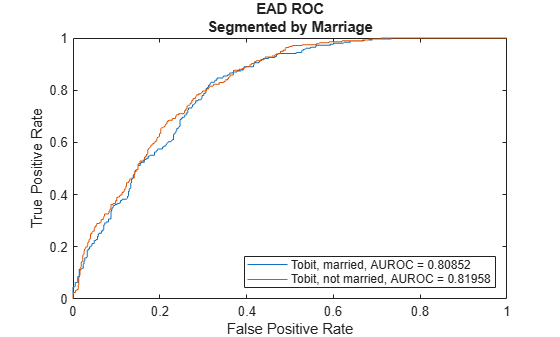This example shows how to use `fitEADModel` to create a `Beta` model and then use `modelDiscriminationPlot` to plot the ROC.

```load EADData.mat head(EADData)```
``` UtilizationRate Age Marriage Limit Drawn EAD _______________ ___ ___________ __________ __________ __________ 0.24359 25 not married 44776 10907 44740 0.96946 44 not married 2.1405e+05 2.0751e+05 40678 0 40 married 1.6581e+05 0 1.6567e+05 0.53242 38 not married 1.7375e+05 92506 1593.5 0.2583 30 not married 26258 6782.5 54.175 0.17039 54 married 1.7357e+05 29575 576.69 0.18586 27 not married 19590 3641 998.49 0.85372 42 not married 2.0712e+05 1.7682e+05 1.6454e+05 ```
```rng('default'); NumObs = height(EADData); c = cvpartition(NumObs,'HoldOut',0.4); TrainingInd = training(c); TestInd = test(c);```

Select Model Type

Select a model type for `Beta`.

`ModelType =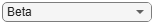"Beta";`

Select Conversion Measure

Select a conversion measure for the EAD response values.

`ConversionMeasure ="LCF";`

Create `Beta` EAD Model

Use `fitEADModel` to create a `Beta` model using the `TrainingInd` data.

```eadModel = fitEADModel(EADData(TrainingInd,:),ModelType,PredictorVars={'UtilizationRate','Age','Marriage'}, ... ConversionMeasure=ConversionMeasure,DrawnVar="Drawn",LimitVar="Limit",ResponseVar="EAD"); disp(eadModel);```
``` Beta with properties: BoundaryTolerance: 1.0000e-07 ModelID: "Beta" Description: "" UnderlyingModel: [1x1 risk.internal.credit.BetaModel] PredictorVars: ["UtilizationRate" "Age" "Marriage"] ResponseVar: "EAD" LimitVar: "Limit" DrawnVar: "Drawn" ConversionMeasure: "lcf" ```

Display the underlying model. The underlying model's response variable is the transformation of the EAD response data. Use the `'LimitVar'` and `'DrawnVar'` name-value arguments to modify the transformation.

`disp(eadModel.UnderlyingModel);`
```Beta regression model: logit(EAD_lcf) ~ 1_mu + UtilizationRate_mu + Age_mu + Marriage_mu log(EAD_lcf) ~ 1_phi + UtilizationRate_phi + Age_phi + Marriage_phi Estimated coefficients: Estimate SE tStat pValue __________ _________ ________ __________ (Intercept)_mu -0.65566 0.11484 -5.7093 1.2616e-08 UtilizationRate_mu 1.7014 0.078094 21.787 0 Age_mu -0.0055901 0.0027603 -2.0252 0.04295 Marriage_not married_mu -0.012576 0.052098 -0.2414 0.80927 (Intercept)_phi -0.50131 0.094625 -5.2979 1.2687e-07 UtilizationRate_phi 0.39731 0.066707 5.956 2.9305e-09 Age_phi -0.001167 0.0023161 -0.50388 0.61439 Marriage_not married_phi -0.013275 0.042627 -0.31143 0.7555 Number of observations: 2627 Log-likelihood: -3140.21 ```

EAD prediction operates on the underlying compact statistical model and then transforms the predicted values back to the EAD scale. You can specify the `predict` function with different options for the `'ModelLevel'` name-value argument.

```predictedEAD = predict(eadModel,EADData(TestInd,:),ModelLevel="ead"); predictedConversion = predict(eadModel,EADData(TestInd,:),ModelLevel="ConversionMeasure");```

For model validation, use `modelDiscrimination`, `modelDiscriminationPlot`, `modelCalibration`, and `modelCalibrationPlot`.

Use `modelDiscrimination` and then `modelDiscriminationPlot` to plot the ROC curve.

```ModelLevel =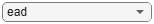"ead"; [DiscMeasure1,DiscData1] = modelDiscrimination(eadModel,EADData(TestInd,:),ModelLevel=ModelLevel); modelDiscriminationPlot(eadModel,EADData(TestInd,:),ModelLevel=ModelLevel,SegmentBy="Marriage");```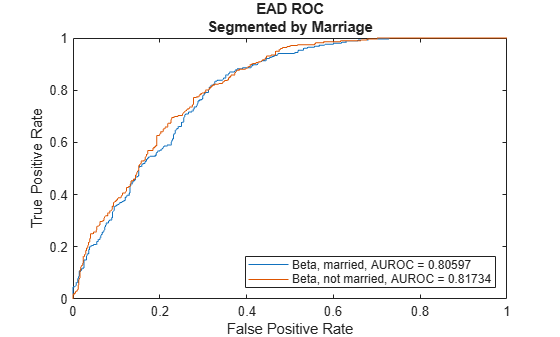## Input Arguments

collapse all

Exposure at default model, specified as a previously created `Regression`, `Tobit`, or `Beta` object using `fitEADModel`.

Data Types: `object`

Data, specified as a `NumRows`-by-`NumCols` table with predictor and response values. The variable names and data types must be consistent with the underlying model.

Data Types: `table`

(Optional) Valid axis object, specified as an `ax` object that is created using `axes`. The plot will be created in the axes specified by the optional `ax` argument instead of in the current axes (gca). The optional argument `ax` must precede any of the input argument combinations.

Data Types: `object`

### Name-Value Arguments

Specify optional pairs of arguments as `Name1=Value1,...,NameN=ValueN`, where `Name` is the argument name and `Value` is the corresponding value. Name-value arguments must appear after other arguments, but the order of the pairs does not matter.

Example: `modelDiscriminationPlot(eadModel,data(TestInd,:),DataID='Testing',DiscretizeBy='median')`

Data set identifier, specified as `DataID` and a character vector or string. The `DataID` is included in the output for reporting purposes.

Data Types: `char` | `string`

Discretization method for EAD `data` at the defined `ModelLevel`, specified as `DiscretizeBy` and a character vector or string.

• `'mean'` — Discretized response is `1` if observed EAD is greater than or equal to the mean EAD, `0` otherwise.

• `'median'` — Discretized response is `1` if observed EAD is greater than or equal to the median EAD, `0` otherwise.

Data Types: `char` | `string`

Name of a column in the `data` input, not necessarily a model variable, to be used to segment the data set, specified as `SegmentBy` and a character vector or string. One AUROC is reported for each segment, and the corresponding ROC data for each segment is returned in the optional output.

Data Types: `char` | `string`

Model level, specified as `ModelLevel` and a character vector or string.

Note

`Regression` models support all three model levels, but a `Tobit` or `Beta` model supports model levels only for `"ead"` and `"conversionMeasure"`.

Data Types: `char` | `string`

EAD values predicted for `data` by the reference model, specified as `ReferenceEAD` and a `NumRows`-by-`1` numeric vector. The ROC curve is plotted for both the `eadModel` object and the reference model.

Data Types: `double`

Identifier for the reference model, specified as `ReferenceID` and a character vector or string. `'ReferenceID'` is used in the plot for reporting purposes.

Data Types: `char` | `string`

## Output Arguments

collapse all

Figure handle for the line objects, returned as handle object.

collapse all

### Model Discrimination Plot

The `modelDiscriminationPlot` function plots the receiver operator characteristic (ROC) curve.

The `modelDiscriminationPlot` function also shows the area under the receiver operator characteristic (AUROC) curve, sometimes called simply the area under the curve (AUC). This metric is between 0 and 1 and higher values indicate better discrimination.

A numeric prediction and a binary response are needed to plot the ROC and compute the AUROC. For EAD models, the predicted EAD is used directly as the prediction. However, the observed EAD must be discretized into a binary variable. By default, observed EAD values greater than or equal to the mean observed EAD are assigned a value of 1, and values below the mean are assigned a value of 0. This discretized response is interpreted as "high EAD" vs. "low EAD." The ROC curve and the AUROC curve measure how well the predicted EAD separates the "high EAD" vs. the "low EAD" observations. You can change the level to compute the model discrimination with the `ModelLevel` name-value pair argument and the discretization criterion with the `DiscretizeBy` name-value pair argument.

The ROC curve is a parametric curve that plots the proportion of

• High EAD cases with predicted EAD greater than or equal to a parameter t, or true positive rate (TPR)

• Low EAD cases with predicted EAD greater than or equal to the same parameter t, or false positive rate (FPR)

The parameter t sweeps through all the observed predicted EAD values for the given data. If the AUROC value or the ROC curve data are needed programmatically, use the `modelDiscrimination` function. For more information about ROC curves, see ROC Curve and Performance Metrics.

 Baesens, Bart, Daniel Roesch, and Harald Scheule. Credit Risk Analytics: Measurement Techniques, Applications, and Examples in SAS. Wiley, 2016.

 Bellini, Tiziano. IFRS 9 and CECL Credit Risk Modelling and Validation: A Practical Guide with Examples Worked in R and SAS. San Diego, CA: Elsevier, 2019.

 Brown, Iain. Developing Credit Risk Models Using SAS Enterprise Miner and SAS/STAT: Theory and Applications. SAS Institute, 2014.

 Roesch, Daniel and Harald Scheule. Deep Credit Risk. Independently published, 2020.